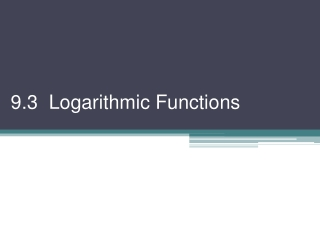DownloadDownload Presentation9.3 Logarithmic Functions

# 9.3 Logarithmic Functions

Télécharger la présentation## 9.3 Logarithmic Functions

- - - - - - - - - - - - - - - - - - - - - - - - - - - E N D - - - - - - - - - - - - - - - - - - - - - - - - - - -
##### Presentation Transcript

1. 9.3 Logarithmic Functions

2. f (x) = bx is one-to-one so it has an inverse. The inverse of an exponential function is called a logarithmic function. For positive real numbers x and b, b > 0 and b ≠ 1, D = x > 0 R =  Do “around the world” start with base inverses • D =  • R = y > 0 • To solve a logarithmic equation, it is often best to start by changing it to its exponential equivalent. • Ex 1) Solve for x • log7x = 2 • 72 = x • 49 = x c) logx 81 = 4 x4 = 81 x = 3 b)

3. Ex 2) Graph. Find Domain, Range, x- & y-int, asymptote, inc or dec. a) f (x) = log2x b) • y = log2x • 2y = x • Plug values into y x y x y –2 2–2= ¼ 4 –2 2–1= ½ –1 2 –1 20 = 1 0 1 0 2 21 = 1 ½ 1 22 = 2 4 ¼ 2 D: x > 0, D: x > 0, R: , R: , x-int: (1, 0), y-int: none, x-int: (1, 0), y-int: none, asympt: x = 0, decreasing asympt: x = 0, increasing *Note: For f (x) = logbx if b > 1, f (x) increases if 0 < b < 1, f (x) decreases!

4. The base of a log function can be any positive number except 1. But, there are two popular & powerful common bases. These have MANY applications to science & engineering (we’ll see tomorrow) Basic Log Facts: (common log) and (natural log) (written as log x) (written as lnx)

5. Ex 3) Simplify each expression. log6 1 0 (log fact!) log3 81 log3 34 4 lne3 logee3 3 e) undefined why??? d) log10 10–2 –2 f) eln6 eloge6 6

6. Homework #903 9-3 Logarithmic Functions WS